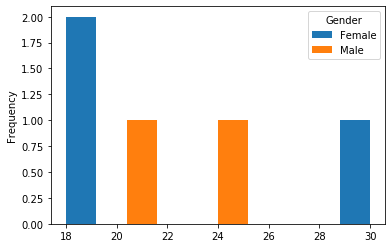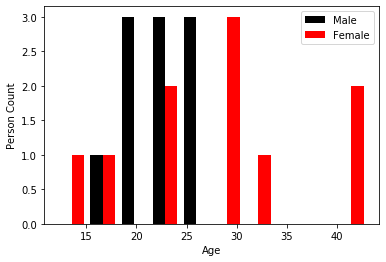GeeksforGeeks App
Open AppBrowser
Continue

# How to fill color by groups in histogram using Matplotlib?

In this article, we are going to see how to fill color by the group in the histogram using Matplotlib in Python.

### Method 1: Using pivot() method

In this method, we are going to use the pivot method which returns an organized DataFrame based on specified index/column values.

Syntax: DataFrameName.pivot(index=’indexLabel’, columns=’columnLabel’, values=’columnName’)

Note: This approach is followed when we want to perform further grouping on the given data.

We use this DataFrame in this approach to plot a histogram representing ages between different genders.

## Python3

 `# import necessary packages``import` `pandas as pd``import` `matplotlib.pyplot as plt` `# create a DataFrame``personAges ``=` `pd.DataFrame({``'Gender'``: [``'Male'``, ``'Female'``,``                                      ``'Male'``, ``'Female'``,``                                      ``'Female'``],``                           ``'Age'``: [``25``, ``19``, ``21``, ``30``, ``18``]})` `# group data & plot histogram``personAges.pivot(columns``=``'Gender'``, values``=``'Age'``).plot.hist()``plt.show()`

Output:Here the original DataFrame is reorganized the Age values based on the Gender column into a new DataFrame. To this reorganized DataFrame we plotted histogram.

The resultant plot indicates there are 2 females between the age 18-20, 1 female is in the age 29-30 and 1 male between 20-22 i.e., 21 and finally 1 male is between 24-25.

### Method 2: Using separated dataset

This method can be followed when we already have separated data on any basis. Let’s consider 2 objects that hold the age of males and females.

```mens_age=18,19,20,21,22,23,24,25,26,27
female_age=22,28,30,30,12,33,41,22,43,18```

If we have the above kind of separated data then we can specify different colors to the different groups while plotting histogram i.e., in hist method.

## Python3

 `# import necessary libraries``import` `matplotlib.pyplot as plt` `mens_age ``=` `18``, ``19``, ``20``, ``21``, ``22``, ``23``, ``24``, ``25``, ``26``, ``27``female_age ``=` `22``, ``28``, ``30``, ``30``, ``12``, ``33``, ``41``, ``22``, ``43``, ``18` `plt.hist([mens_age, female_age], color``=``[``         ``'Black'``, ``'Red'``], label``=``[``'Male'``, ``'Female'``])``plt.xlabel(``'Age'``)``plt.ylabel(``'Person Count'``)``plt.legend()``plt.show()`

Output:As we already have grouped data based on gender we no need to use any pivot function. So we can directly specify those separate sets of data with different colors in hist function.

These are the two approaches that need to be followed based on the given data and requirement to fill color by groups in the histogram.

My Personal Notes arrow_drop_up